# JEE Main Mathematics Mock - 2

## 25 Questions MCQ Test Mock Test Series for JEE Main & Advanced 2022 | JEE Main Mathematics Mock - 2

Description
Attempt JEE Main Mathematics Mock - 2 | 25 questions in 60 minutes | Mock test for JEE preparation | Free important questions MCQ to study Mock Test Series for JEE Main & Advanced 2022 for JEE Exam | Download free PDF with solutions
QUESTION: 1

Solution:
QUESTION: 2

### If the equation [(k(x+1)2/3)]+[(y+2)2/4]=1 represents a circle, then k=

Solution: The given equation can be write as

=> 4k(x+1) ²+3(y+2) ²=12

on expanding wee get x² coefficient as 4k
and y² coefficient as 3
but in equation of circle x² coefficient is equal to y² coefficient

therefore 4k=3
=> k=3/4
QUESTION: 3

### The eccentric angles of the extremities of the latus-rectum intersecting positive x-axis of the ellipse ((x2/a2) + (y2/b2) = 1) are given by

Solution:
QUESTION: 4

If arg (z) = θ, then arg(z̅) =

Solution:
QUESTION: 5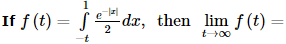Solution:
QUESTION: 6

If N N+ denotes the set of all positive integers and if f : NN+ → N is defined by f(n)    = the sum of positive divisors of (n)  then f (2k . 3), where k is a positive integer is

Solution:

f(2k. 3) = The sum of positive divisors of 2k . 3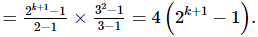QUESTION: 7

If a, b, c are different and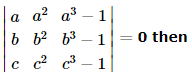Solution:

Explanation : A = {(a, a2, a3-1) (b, b2, b3-1) (c, c2, c3-1)}

=> {(a, a2, a3) (b, b2, b3) (c, c2, c3)} - {(a, a2, 1) (b, b2, 1) (c, c2, 1)} = 0

=> abc{(1, a, a2) (1, b, b2) (1, c, c2)} - {(a, a2, 1) (b, b2, 1) (c, c2, 1)} = 0

=> abc{(a, a2, 1) (b, b2, 1) (c, c2, 1)} - {(a, a2, 1) (b, b2 1) (c, c2, 1)} = 0

=> (abc-1){(a, a2, 1) (b, b2, 1) (c, c2, 1)} = 0

abc - 1 = 0

=> abc = 1

QUESTION: 8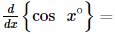Solution: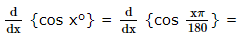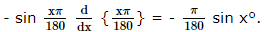QUESTION: 9

In the following question, a Statement of Assertion (A) is given followed by a corresponding Reason (R) just below it. Read the Statements carefully and mark the correct answer-
Assertion (A): Angle between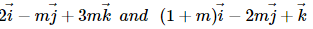is acute angle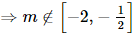Reason (R): If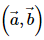is acute then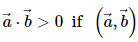is obtuse then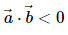Solution:
QUESTION: 10

The point on the curve y = x2 which is nearest to (3, 0) is

Solution:
QUESTION: 11

The degree of the differential equation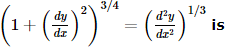Solution: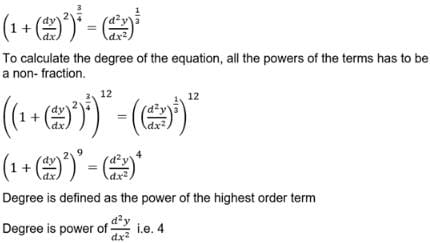QUESTION: 12

In the following question, a Statement of Assertion (A) is given followed by a corresponding Reason (R) just below it. Read the Statements carefully and mark the correct answer-
Assertion (A):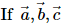are non zero vectors then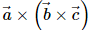is a vector perpendicular to all the vectors a → , b → , c →
Reason (R):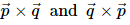are perpendicular to both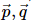Solution:
QUESTION: 13

If i2 = -1, then the sum i + i2 + i3 + ..... upto 1000 terms is equal to

Solution: There will equal n opposite signed terms that is 500 +ve one and 500-ve one therefore it's value comes to zero.
QUESTION: 14

A parallelogram is cut by two sets of m lines parallel to the sides, the number of parallelogram thus formed is

Solution:
Parallelogram is cut by two sets of m parallel lines to its sides.
then we have  2 sets of (m+2) parallel lines ( 2 lines of the parallelogram)
so parallelogram is formed by taking 2 lines from each set
= m+2C2 * m+2C2
= [(m+2)(m+1)/2 ]2
this also include 1 original parallelogram
so total number of new parallelogram formed is  =  (m + 2)2(m + 1)2/4
QUESTION: 15

If A and B are two events such that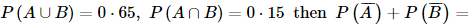Solution:
QUESTION: 16

The probability that a leap year will have exactly 52 Tuesdays is

Solution:

The probability of a year being a leap year is 1/4 and being non-leap is 3/4.A leap year has 366 days or 52 weeks and 2 odd days. The two odd days can be {Sunday,Monday},{Monday,Tuesday},{Tuesday,Wednesday}, Wednesday,Thursday},{Thursday,Friday},{Friday,Saturday},{Saturday,Sunday}.So there are 7 possibiliyies out of which 2 have a Sunday. So the probability of 53 Sundays in a leap year is 2/7.

So, the probability of 52 sundays is 1-2/7 = 5/7.

QUESTION: 17

Product of the real roots of the equation t2x2 + ∣x∣ + 9 = 0

Solution:
QUESTION: 18

If A.M. between two numbers is 5 and their G.M. is 4, then their H.M. is

Solution:

If x, y and z respectively represent AM, GM and HM between two numbers a and b, then
y2 = xz
Here x = 5, y = 4
then 16 = 5 x z
z = 16/5

QUESTION: 19

If the coefficient of correlation between x and y is 0.28, covariance between x and y is 7.6, and the variance of x is 9, then the standard deviation of the y series is

Solution: N the given problem it is SD of x is 3: (or Variance of x is 9). As Variance = (Sx)^2. We know the relation : correlation coefficient (r) = Cov (x,y) / (Sx * Sy) so, 0.28 = 7.6 / (3 * Sy) From here we get the value of SD of Y : Sy = 9.05.
QUESTION: 20

The equation line passing through the point P(1,2) whose portion cut by axes is bisected at P, is

Solution: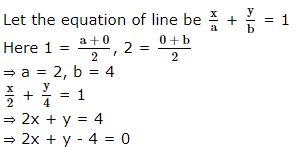*Answer can only contain numeric values
QUESTION: 21

If 2 tan2x – 5 sec x is equal to 1 for exactly 7 distinct values of X ∈ [0, nπ/2], n ∈ N, then the greatest value of n is

Solution:

2tan2x – 5sec x = 1
2 (sec2x – 1) – 5secx = 1
2sec2x – 5sec – 3 = 1
∴  cosx = 1/3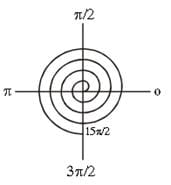*Answer can only contain numeric values
QUESTION: 22

If the mean deviation of the number 1, 1 + d, ... , 1 + 8d from their mean is 205, then d is equal to

Solution: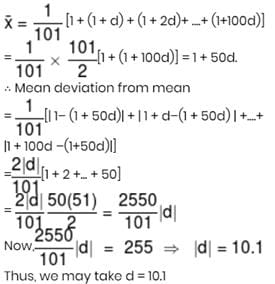*Answer can only contain numeric values
QUESTION: 23

The number of 5-digit numbers of the form xyzyx in which x < y is :-

Solution:

for z → 10 choice
for  First two x and y → 9C2 choice
Last two y and x → 1 choice
10 × 9C2 × 1= 360

*Answer can only contain numeric values
QUESTION: 24

If z1 and z2 are two unimodular complex numbers that satisfy z12 + z22 = 5, then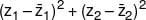is equal to -

Solution: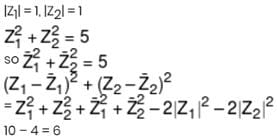*Answer can only contain numeric values
QUESTION: 25

The AM of 9 term is 15. If one more term is added to this series, then the A.M. becomes 16. The value of added term is :

Solution:

Sum of 9 term = 9 × 15 = 135
New sum when Mean is 16
= 16 × 10 = 160
New term = 160 – 135 ⇒ 25Use Code STAYHOME200 and get INR 200 additional OFF Use Coupon Code